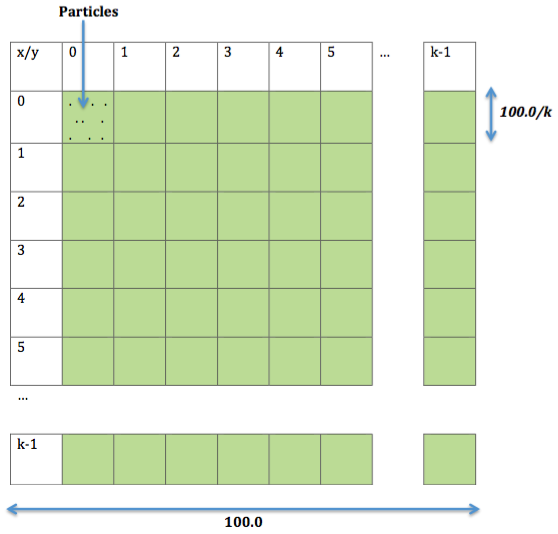# Chare Arrays: Particle ExerciseFigure 1

## Random Migrating Particles

For this exercise, you will write code that simulates a set of particles moving randomly in a 2-dimensional space within a bounding box. The coordinates of the overall simulation box are between 0.0 and 100.0 along each dimension. The particles are divided among chares based on their coordinates. The chares should be organized in a 2-dimensional array of chares (using a chare array) of size k \times k. So, each chare owns a bounding box of its own with size 100.0/k. The particles in each chare can be stored as a vector.

Your program should generate n particles per chare during construction with a random (but valid, i.e. within the chare) position for particles. Your program should accept the number of particles per cell n, and k as command line parameters in that sequence.

Expected Output: Your program should calculate and print to screen the maximum and total number of particles every 10 iterations. Additionally, the simulation should not be delayed by this calculation (i.e. you should use reductions).

For testing your program, you can use 10000 (=n) particles per chare, simulated over 100 steps and a chare array of size 16 \times 16 (k=16). Experiment with different number of particles and chare array sizes.

Note: There might be multiple particles having the same x and y coordinates, especially if you increase the density of each cell. You do not need to handle this case separately; it is a valid case assumption.

• The pseudocode for the overall algorithm is:
• for(iteration=0; iteration<ITERATION; iteration++){
• For each of the particles that belong to my chare: change its x and y coordinate by a small random amount.
• Move all the particles that do not belong to a chare's bounding box to their correct homes. Since the movement is small, this will mean communication to the eight near neighbor chares. Some of these messages may contain no particles.
• if(iteration%10 == 0)
• Do reductions to calculate average and max number of particles
}